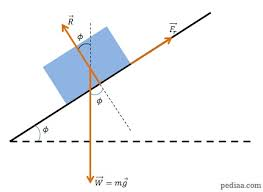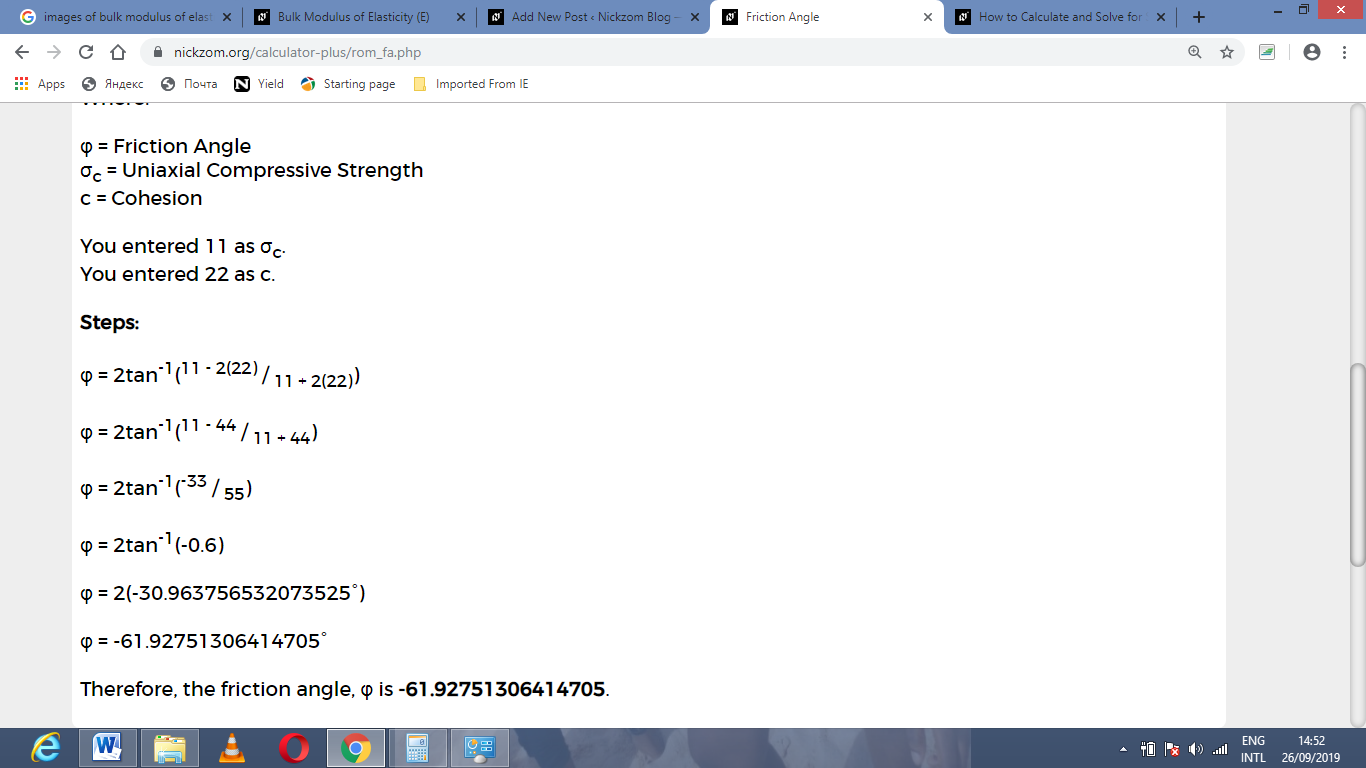# How to Calculate and Solve for Friction Angle | Rock MechanicsThe image above represents friction angle.

To compute for friction angle, two essential parameters are needed and these parameters are Uniaxial Compressive Strength (σc) and cohesion (c).

The formula for calculating the friction angle:

φ = 2tan-1(σc – 2c / σc + 2c)

Where;

φ = Friction Angle
σc = Uniaxial Compressive Strength
c = Cohesion

Let’s solve an example;
Find the friction angle when the uniaxial compressive strength is 11 and the cohesion is 22.

This implies that;

σc = Uniaxial Compressive Strength = 11
c = Cohesion = 22

φ = 2tan-1(σc – 2c / σc + 2c)
φ = 2tan-1(11 – 2(22) / 11 + 2(22))
φ = 2tan-1(11 – 44 / 11 + 44)
φ = 2tan-1(-33 / 55)
φ = 2tan-1(-0.6)
φ = 2(-30.96°)
φ = -61.927

Therefore,  the friction angle is -61.927.

Nickzom Calculator – The Calculator Encyclopedia is capable of calculating the friction angle.

To get the answer and workings of the friction angle using the Nickzom Calculator – The Calculator Encyclopedia. First, you need to obtain the app.

You can get this app via any of these means:

To get access to the professional version via web, you need to register and subscribe for NGN 1,500 per annum to have utter access to all functionalities.
You can also try the demo version via https://www.nickzom.org/calculator

Apple (Paid) – https://itunes.apple.com/us/app/nickzom-calculator/id1331162702?mt=8
Once, you have obtained the calculator encyclopedia app, proceed to the Calculator Map, then click on Geology under Add-on.Now, Click on Rock Mechanics under GeologyNow, Click on Friction Angle under Rock MechanicsThe screenshot below displays the page or activity to enter your values, to get the answer for the friction angle according to the respective parameters which are the Uniaxial Compressive Strength (σc) and cohesion (c).Now, enter the values appropriately and accordingly for the parameters as required by the Uniaxial Compressive Strength (σc) is 11 and cohesion (c) is 22.Finally, Click on CalculateAs you can see from the screenshot above, Nickzom Calculator– The Calculator Encyclopedia solves for the friction angle and presents the formula, workings and steps too.